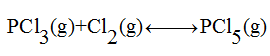# A student placed 0.200 mol of PCl3(g) and 0.100 mol of Cl2(g) into a 1.00 liter container at 250  oC. After the reaction.PCl3(g) + Cl2(g)↔PCl5(g) came to equilibrium, it was found that the flask contained 0.120 mol of PCl3. What is the value of K for this reaction?

Question
167 views
A student placed 0.200 mol of PCl3(g) and 0.100 mol of Cl2
(g) into a 1.00 liter container at 250  oC. After the reaction.
PCl3(g) + Cl2(g)↔PCl5(g) came to equilibrium, it was found that the flask contained 0.120 mol of PCl3. What is the value of K for this reaction?
check_circle

Step 1

The reaction is formation of phosphorous pentachloride.Given:

Volume of container = 1 L

Initial moles of PCl3(g) = 0.200 mol

Initial moles of Cl2(g) =0.100 mol

Equilibrium moles of PCl5(g) = 0.120

As volume is one litre we can consider moles as concentration also.

Step 2

As per given equation, one mole of PCl3 will react with one mole of Cl2 to given one mole of PCl5.

Let the concentration decrease in PCl3 to reach equilibrium = x

So the decrease in concentration of Cl2 will also be = x

Hence the concentration of PCl5 formed at equilibrium = x

We are given with the moles of PCl3 at equilibrium = 0.120

So x = 0.200-0.120 = 0.080

Step 3

We can also understand it in terms ...

### Want to see the full answer?

See Solution

#### Want to see this answer and more?

Solutions are written by subject experts who are available 24/7. Questions are typically answered within 1 hour.*

See Solution
*Response times may vary by subject and question.
Tagged in

### Chemistry